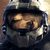0
Not a bug

# subroutine precision

dattorro 1 year ago updated by Pavel Holoborodko 1 year ago

"If input parameters a and b are multiprecision type, then all subsequent calculations inside the function will be conducted with high precision,..."

Re: Matlab 2021a under Windows 10:
User-written subroutines are executed in quadruple precision (34 digits) regardless of number of digits possessed by arguments passed to the subroutine.

For example: If arg1 has 100-digit precision and is passed to subroutine1(arg1), then computations within subroutine1 will be performed in 34-digit precision unless the following statement appears inside:

mp.Digits(mp.Digits(arg1));Not a bugWe do not recommend to mix different precisions. That is because sematic of mixed-precision computations are not well posed/defined. Do we have to use maximum precision of two arguments, or minimum, or average or else? That is a long-standing and non-resolved question in scientific community.

If you want to compare different precisions, then do all computations using one precision, store results for each precision (mat/txt file). And finally use the highest precision to load and compare them.

For example, assuming B>A:

Setup mp.Digits(A), run all the computations and store final results for precision A. Then setup mp.Digits(B) and re-run all computations from the start and store results for precision B. Setup mp.Digits(B), load all the results from files, and compare results (do not re-run the computations).

"User-written subroutines are executed in quadruple precision (34 digits) regardless of number of digits possessed by arguments passed to the subroutine."

Yes, this is true, if all expressions in subroutine use only arguments, e.g. r = a+b.

But, if expressions use some constants, e.g. r = sin(mp('pi')*x) - the final precision will be different. Because toolbox uses default precision to convert entities of "unknown" precision, like double numbers. So that if your default precision is mp.Digits(34), then it will be used for mp('pi'), regardless of what precision x has.We are not suggesting mixed precision.

My original query concerns only passing number-of-digits precision to a subroutine.

User-written subroutines do not respect precision of the arguments;

i.e, the subroutine defaults to 34 digits regardless of input argument precision.

```>> mp.Digits(100);
>> x = mp.rand(100,100);
>> norm(x-ifft(fft(x)),1)
3.1e-103

>> tic; ifft(fft(x)); toc;
Elapsed time is 0.839798 seconds.```

The fft() respects input precision of the arguments.
This leads to an expectation of consistency; i.e, a subroutine (user-written or otherwise) should always be executed in precision of the arguments.  But that is not the case.

Pavel, where is the treatment of subroutine execution precision disclosed in the documentation?  I cannot find it.@"The fft() respects input precision of the arguments."
All functions provided in toolbox respect precision of the input arguments.

However, user-written functions might behave differently - this is responsibility of the user who writes the code. See my previous reply for the cases when precision might be different inside user-written function (e.g. when mix of different precisions is used).

Could you please provide minimal, complete, and verifiable example of the improper behavior of the toolbox?

This would help a lot.

Edit:

@"This leads to an expectation of consistency; i.e, a subroutine (user-written or otherwise) should always be executed in precision of the arguments."

All functions in toolbox provide this consistency. But toolbox has no control of user-written code. All user's M-code is executed by MATLAB interpreter by following the object programming rules (it calls functions from toolbox when it encounters mp-variables). We cannot change this and force the MATLAB interpreter to behave differently.

In order to have this consistency in user-written functions, please use the same working precision everywhere. This is the only simple rule.